#.

Pandas dataframe is making life a lot easier if you are working with data. A lot of data comes in CSV format. It's possible to read CSV files to a Pandas dataframe. In fact, it's quite easy using read_csv. In the video below we will learn the basics of just loading a CSV file to a Pandas dataframe object.

There are many more ways to work with the Pandas read_csv(). The following is an article originally posted method to here.

In the first section, we will go through, with examples, how to read a CSV file, how to read specific columns from a CSV, how to read multiple CSV files and combine them to one dataframe, and, finally, how to convert data according to specific datatypes (e.g., using Pandas read_csv dtypes).

In the last section, we will continue by learning how to use Pandas to write CSV files. That is, we will learn how to export dataframes to CSV files.

## Pandas Import CSV from the Harddrive

In the first example, of this Pandas read CSV tutorial, we will just use read_csv to load CSV to dataframe that is in the same directory as the script. If we have the file in another directory we have to remember to add the full path to the file.

Naturally, Pandas can be used to import data from a range of different file types. For instance, Excel (xlsx), and JSON files can be read into Pandas dataframes. Learn more about importing data in Pandas:

### How to Read CSV File in Python Pandas

In this section, we are going to learn how to read CSV files from the harddrive. First, however, we are going to answer the question How do I open a CSV file in pandas?

### How do I import a CSV file into Pandas using Python?

Here’s two simple steps to learn how to read a CSV file in Pandas:

1. Import the Pandas package:
`import pandas as pd`

`df = pd.read_csv('yourCSVfile.csv')`
Note, the first argument of the read_csv method should be the file path to your CSV file.

In this Pandas read CSV tutorial, we will learn how to work with comma-separated (CSV) files in Python and Pandas. We will get an overview of how to use Pandas to load CSV to dataframes and how to write dataframes to CSV.

``df = pd.read_csv('amis.csv') df.head()``

If we are interested in reading files, in general, using Python we can use the open() method. This way, we can read many file formats (e.g., .txt) in Python.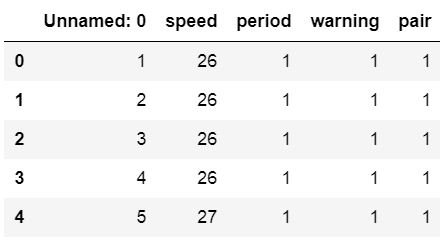https://www.marsja.se/wp-content/uploads/2018/11/pandas-read-csv-to-dataframe-example-300x165.jpg 300w" sizes="(max-width: 446px) 100vw, 446px" />
Dataframe

The data can be downloaded here but in the following examples, we are going to use Pandas read_csv to load data from a URL.

## Pandas Read CSV from a URL

Can we import a CSV file from a URL using Pandas? Yes, and in this section, we are going to learn how to read a CSV file in Python using Pandas, just like in the previous example. However, in the next read_csv example, we are going to read the same dataset but this time from a URL. It’s very simple we just put the URL in as the first parameter in the read_csv method. Here’s three simple steps that will help us read a CSV from a URL:

1. Again, we need to import Pandas
2. Create a string variable with the URL
3. Now use Pandas read_csv together with the URL (see example below)

In the example code below, we follow the three easy steps to import a CSV file into a Pandas dataframe:

``url_csv = 'https://vincentarelbundock.github.io/Rdatasets/csv/boot/amis.csv' df = pd.read_csv(url_csv) df.head()``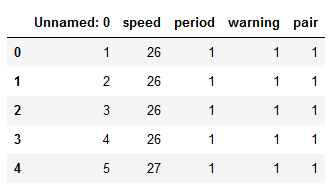https://www.marsja.se/wp-content/uploads/2018/11/how_to_read_csv_file_pandas-300x173.png 300w" sizes="(max-width: 335px) 100vw, 335px" />

In the image above, we can see that we get a column named ‘Unnamed: 0’. Furthermore, we can see that it contains numbers. Thus, when using Pandas read_csv method, we can use this column as the index column. In the next code example, we are doing exactly this; we are going to use Pandas read_csv and the index_col parameter.

This parameter can take an integer or a sequence. In our case, we are going to use the integer 0 and we will get a nicer dataframe:

``df = pd.read_csv(url_csv, index_col=0) df.head()``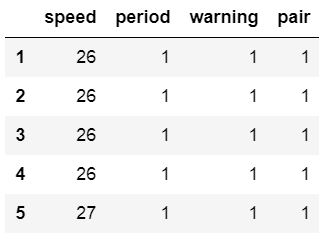https://www.marsja.se/wp-content/uploads/2018/11/pandas-read-csv-example-300x222.jpg 300w, https://www.marsja.se/wp-content/uploads/2018/11/pandas-read-csv-ex... 203w" sizes="(max-width: 331px) 100vw, 331px" />

The index_col parameter also can take a string as input and we will now use a different datafile. In the next example we will read a CSV into a Pandas dataframe and use the idNum column as index.

``csv_url = 'http://vincentarelbundock.github.io/Rdatasets/csv/carData/MplsStops.csv' df = pd.read_csv(csv_url, index_col='idNum') df.iloc[:, 0:6].head()``https://www.marsja.se/wp-content/uploads/2018/11/pandas-read-csv-index-cols-multiindex-300x102.jpg 300w, https://www.marsja.se/wp-content/uploads/2018/11/pandas-read-csv-in... 640w, https://www.marsja.se/wp-content/uploads/2018/11/pandas-read-csv-in... 879w" sizes="(max-width: 768px) 100vw, 768px" />

Note, to get the above output we used Pandas iloc to select the first 7 rows. This was done to get an output that could be easier illustrated. That said, we are now continuing to the next section where we are going to read certain columns to a dataframe from a CSV file.

In some cases, we don’t want to parse every column in the CSV file. To only read certain columns we can use the parameter usecols. Note, if we want the first column to be index column and we want to parse the three first columns we need to have a list with 4 elements (compare my read_excel usecols example here):

``cols = [0, 1, 2, 3] df = pd.read_csv(url_csv,                    index_col=0, usecols=cols) df.head()``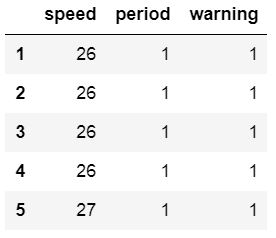Of course, using read_csv usecols make more sense if we had a CSV file with more columns. We can use Pandas read_csv usecols with a list of strings, as well. In the next example we return to the larger file we used previously:

``csv_url = 'http://vincentarelbundock.github.io/Rdatasets/csv/carData/MplsStops.csv' df = pd.read_csv(csv_url, index_col='idNum',                    usecols=['idNum', 'date', 'problem', 'MDC']) df.head()``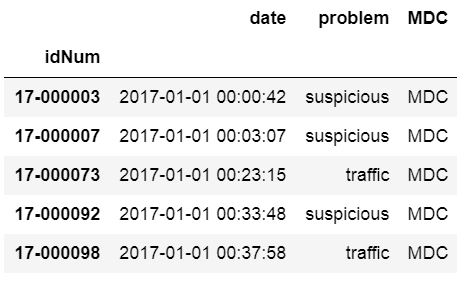https://www.marsja.se/wp-content/uploads/2018/11/pandas-read-csv-usecols-example-1-300x185.jpg 300w" sizes="(max-width: 466px) 100vw, 466px" />
usecols with list of strings

## Pandas Read CSV: Remove Unnamed Column

In some of the previous read_csv example, we get an unnamed column. In previous sections, of this Pandas read CSV tutorial, we have solved this by setting this column as index or used usecols to select specific columns from the CSV file. However, we may not want to do that for some reason. Here’s one example of how to use Pandas read_csv to get rid of the column “Unnamed:0”:

``csv_url = 'http://vincentarelbundock.github.io/Rdatasets/csv/carData/MplsStops.csv' cols = pd.read_csv(csv_url, nrows=1).columns df = pd.read_csv(csv_url, usecols=cols[1:]) df.iloc[:, 0:6].head()``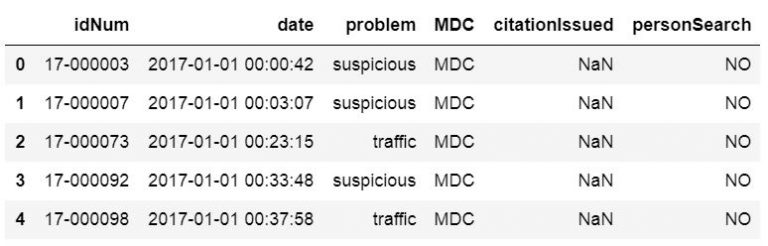https://www.marsja.se/wp-content/uploads/2018/11/pandas-read-csv-tutorial-300x96.jpg 300w, https://www.marsja.se/wp-content/uploads/2018/11/pandas-read-csv-tu... 640w, https://www.marsja.se/wp-content/uploads/2018/11/pandas-read-csv-tu... 774w" sizes="(max-width: 768px) 100vw, 768px" />

### How to Drop a Column from Pandas dataframe

It’s of course also possible to remove the unnamed columns after we have loaded the CSV to a dataframe. To remove the unnamed columns we can use two different methods; loc and drop, together with other Pandas dataframe methods. When using the drop method we can use the inplace parameter and get a dataframe without unnamed columns.

``df.drop(df.columns[df.columns.str.contains('unnamed', case=False)],           axis=1, inplace=True)  # The following line will give us the same result as the line above # df = df.loc[:, ~df.columns.str.contains('unnamed', case=False)]  df.iloc[:, 0:7].head()``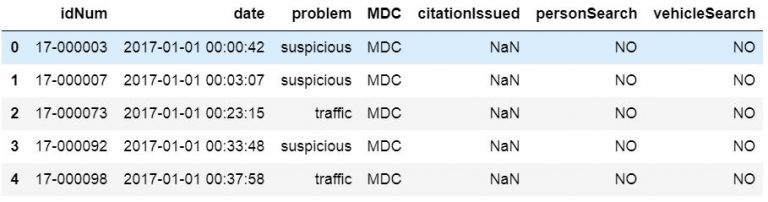https://www.marsja.se/wp-content/uploads/2018/11/removing-many-unnamed-columns-from-pandas-dataframe-300x79.jpg 300w, https://www.marsja.se/wp-content/uploads/2018/11/removing-many-unna... 640w, https://www.marsja.se/wp-content/uploads/2018/11/removing-many-unna... 909w" sizes="(max-width: 768px) 100vw, 768px" />

To explain the code example above; we select the columns without columns containing the string ‘unnamed’. Furthermore, we used the case parameter so that the contains method is not case-sensitive. Thus, we will get columns named “Unnamed” and “unnamed”. In the first row, using Pandas drop, we are also using the inplace parameter so that it changes our dataframe. The axis parameter, however, is used to drop columns instead of indices (i.e., rows).

## Pandas Read CSV and Missing Values

In this section, we will learn how to handle missing values in a Pandas dataframe. If we have missing data in our CSV file and it’s coded in a way that makes it impossible for Pandas to find them we can use the parameter na_values. In the example below the amis.csv file has been changed and there are some cells with the string “Not Available”.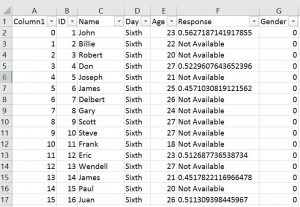https://www.marsja.se/wp-content/uploads/2018/11/missing-data-pandas-dataframe-768x529.jpg 768w, https://www.marsja.se/wp-content/uploads/2018/11/missing-data-panda... 640w, https://www.marsja.se/wp-content/uploads/2018/11/missing-data-panda... 782w" sizes="(max-width: 300px) 100vw, 300px" />
CSV file

That is, we are going to change “Not Available” to something that we easily can remove when carrying out data analysis later.

``df = pd.read_csv('Simdata/MissingData.csv', index_col=0,                    na_values="Not Available") df.head()``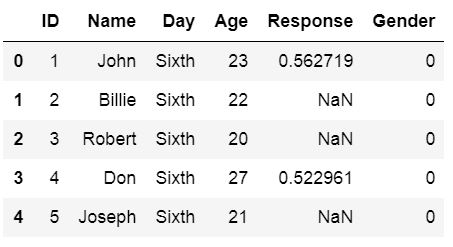https://www.marsja.se/wp-content/uploads/2018/11/read-csv-pandas-missing-values-300x164.jpg 300w" sizes="(max-width: 453px) 100vw, 453px" />

## Reading CSV and Skipping Rows

What if our data file(s) contain information on the first x rows and we need to skip rows when using Pandas read_csv? For instance, how can we skip the first three rows in a file looking like this: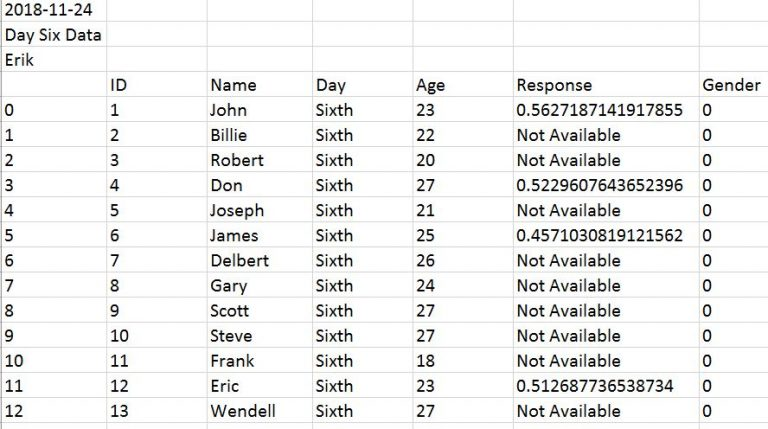https://www.marsja.se/wp-content/uploads/2018/11/read-csv-pandas-skip-rows-300x168.jpg 300w, https://www.marsja.se/wp-content/uploads/2018/11/read-csv-pandas-sk... 640w, https://www.marsja.se/wp-content/uploads/2018/11/read-csv-pandas-sk... 888w" sizes="(max-width: 768px) 100vw, 768px" />

We will now learn how to use Pandas read_csv and skip x amount of rows. Luckily, it’s very simple we just use the skiprows parameter. In the following example, we are using read_csv and skiprows=3 to skip the first 3 rows.

How do we use Pandas skiprow parameter? Here’s a Pandas read_csv example, where we skip the three first rows:

``df = pd.read_csv('Simdata/skiprow.csv', index_col=0, skiprows=3) df.head()``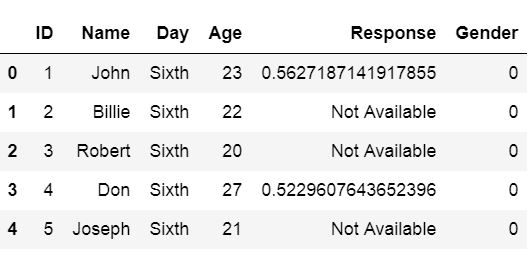https://www.marsja.se/wp-content/uploads/2018/11/skip-rows-pandas-csv-tutorial-300x145.jpg 300w" sizes="(max-width: 532px) 100vw, 532px" />

Note we can obtain the same result as above using the header parameter (i.e., data = pd.read_csv(‘Simdata/skiprow.csv’, header=3)).

## How to Read Certain Rows using Pandas

Can we read specific rows from a CSV file using Pandas read_csv method? If we don’t want to read every row in the CSV file we ca use the parameter nrows. In the next example, below we read the first 8 rows of a CSV file.

``df = pd.read_csv(url_csv, nrows=8) df``

We can also set the data types for the columns. Although, in the amis dataset all columns contain integers we can set some of them to string data type. This is exactly what we will do in the next Pandas read_csv pandas example. We will use the Pandas read_csv dtype parameter and put in a dictionary:

``url_csv = 'https://vincentarelbundock.github.io/Rdatasets/csv/boot/amis.csv' df = pd.read_csv(url_csv, dtype={'speed':int, 'period':str, 'warning':str, 'pair':int}) df.info()``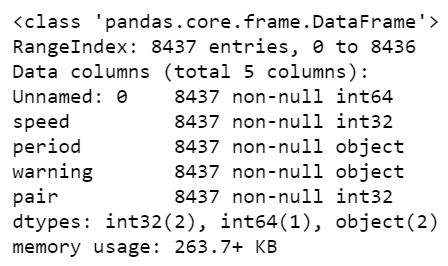https://www.marsja.se/wp-content/uploads/2018/11/pandas-read-csv-dtype-string-300x178.jpg 300w" sizes="(max-width: 448px) 100vw, 448px" />

It’s ,of course, possible to force other datatypes such as integer and float. All we have to do is change str to float, for instance (given that we have decimal numbers in that column, of course).

## Load Multiple Files to a Dataframe

If we have data from many sources such as experiment participants we may have them in multiple CSV files. If the data, from the different CSV files, are going to be analyzed together we may want to load them all into one dataframe. In the next examples, we are going to use Pandas read_csv to read multiple files.

First, we are going to use Python os and fnmatch to list all files with the word “Day” of the file type CSV in the directory “SimData”. Next, we are using Python list comprehension to load the CSV files into dataframes (stored in a list, see the type(dfs) output).

``import os, fnmatch  csv_files = fnmatch.filter(os.listdir('./SimData'), '*Day*.csv') dfs = [pd.read_csv('SimData/' + os.sep + csv_file)        for csv_file in csv_files]  type(dfs) # Output: list``

Finally, we use the method concat to concatenate the dataframes in our list. In the example files, there is a column called ‘Day’ so that each day (i.e., CSV file) is unique.

``df = pd.concat(dfs, sort=False) df.Day.unique()``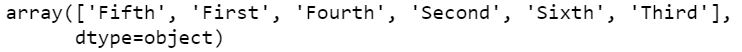https://www.marsja.se/wp-content/uploads/2018/11/read-many-csv-files-to-pandas-dataframe-300x21.jpg 300w, https://www.marsja.se/wp-content/uploads/2018/11/read-many-csv-file... 640w" sizes="(max-width: 742px) 100vw, 742px" />

The second method we are going to use is a bit simpler; using Python glob. If we compare the two methods (os + fnmatch vs. glob) we can see that in the list comprehension we don’t have to put the path. This is because glob will have the full path to our files. Handy!

``import glob  csv_files = glob.glob('SimData/*Day*.csv') dfs = [pd.read_csv(csv_file) for csv_file in csv_files]  df = pd.concat(dfs, sort=False)``

If we don’t have a column, in each CSV file, identifying which dataset it is (e.g., data from different days) we could apply the filename in a new column of each dataframe:

``import glob  csv_files = glob.glob('SimData/*Day*.csv') dfs = []  for csv_file in csv_files:     temp_df = pd.read_csv(csv_file)     temp_df['DataF'] = csv_file.split('\\')     dfs.append(temp_df)``

There are, of course, times when we need to rename multiple files (e.g., CSV files before loading them into Pandas dataframes). Luckily, to rename a file in Python we can use os.rename(). This method can be used regardless if we need to rename CSV or .txt files.

Now we know how to import multiple CSV files and, in the next section, we will learn how to use Pandas to write to a CSV file.

## How to Write CSV files in Pandas

In this section, we will learn how to export dataframes to CSV files. We will start by creating a dataframe with some variables but first, we start by importing the modules Pandas:

``import pandas as pd``

Before we go on and learn how to use Pandas to write a CSV file, we will create a dataframe. We will create the dataframe using a dictionary. The keys will be the column names and the values will be lists containing our data:

``df = pd.DataFrame({'Names':['Andreas', 'George', 'Steve',                            'Sarah', 'Joanna', 'Hanna'],                   'Age':[21, 22, 20, 19, 18, 23]}) df.head()``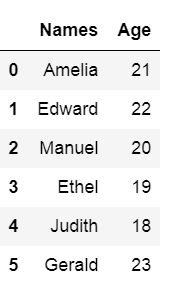### How to Save Pandas to CSV

Now we are ready to learn how to save Pandas dataframe to CSV. It’s quite simple, we write the dataframe to CSV file using Pandas to_csv method. In the example below we don’t use any parameters but the path_or_buf which is, in our case, the file name.

``df.to_csv('NamesAndAges.csv')``

Here’s how the exported dataframe look like: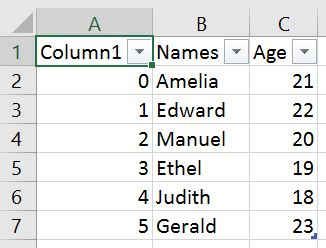https://www.marsja.se/wp-content/uploads/2018/11/pandas-export-dataframe-to-csv-index-column-300x228.jpg 300w" sizes="(max-width: 326px) 100vw, 326px" />

As can be seen in the image above we get a new column when we are not using any parameters. This column is the index column from our Pandas dataframe. When working with Pandas to_csv, we can use the parameter index and set it to False to get rid of this column.

``df.to_csv('NamesAndAges.csv', index=False)``

### How to Write Multiple Dataframes to one CSV file

If we have many dataframes and we want to export them all to the same CSV file it is, of course, possible. In the Pandas to_csv example below we have 3 dataframes. We are going to use Pandas concat with the parameters keys and names.

This is done to create two new columns, named Group and Row Num. The important part is Group which will identify the different dataframes. In the last row of the code example we use Pandas to_csv to write the dataframes to CSV.

``df1 = pd.DataFrame({'Names': ['Andreas', 'George', 'Steve',                            'Sarah', 'Joanna', 'Hanna'],                    'Age':[21, 22, 20, 19, 18, 23]}) df2 = pd.DataFrame({'Names': ['Pete', 'Jordan', 'Gustaf',                            'Sophie', 'Sally', 'Simone'],                    'Age':[22, 21, 19, 19, 29, 21]}) df3 = pd.DataFrame({'Names': ['Ulrich', 'Donald', 'Jon',                            'Jessica', 'Elisabeth', 'Diana'],                    'Age':[21, 21, 20, 19, 19, 22]})   df = pd.concat([df1, df2, df3], keys =['Group1', 'Group2', 'Group3'],                names=['Group', 'Row Num']).reset_index()  df.to_csv('MultipleDfs.csv', index=False)``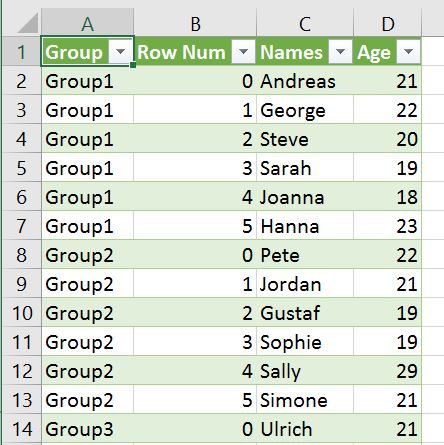https://www.marsja.se/wp-content/uploads/2018/11/save-to-csv-pandas-example-150x150.jpg 150w, https://www.marsja.se/wp-content/uploads/2018/11/save-to-csv-pandas... 300w" sizes="(max-width: 444px) 100vw, 444px" />

In the CSV file we get 4 columns. The keys parameter with the list ([‘Group1’, ‘Group2’, ‘Group3’]) will enable identification of the different dataframes we wrote. We also get the column “Row Num” which will contain the row numbers for each dataframe:

## Conclusion

In this tutorial we have learned about importing CSV files into Pandas dataframe. More specifically, we have learned how to:

• locally
• from the WEB
• Remove unnamed columns
• Handle missing values
• Skipping rows and reading certain rows
• Changing datatypes using dtypes
• Saving dataframes to CSV using Pandas to_csv

Views: 3313

Tags: data, pandas, psychology, python, science

CommentComment by JackDonald34 on April 29, 2021 at 4:44am